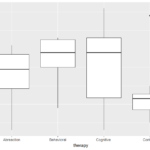Want to share your content on R-bloggers? click here if you have a blog, or here if you don't.In ANOVA our interest lies in knowing if one continuous dependent variable is affected by one or more categorical independent variables. MANOVA is an extension of ANOVA where we are now able to understand how several dependent variables are affected by independent variables. For example consider an investigation where a medical investigator has developed 3 back pain therapies. Patients are enrolled for a 10 week trial and at the end the investigator interviews them on reduction of physiological, emotional and cognitive pain. Interest is in knowing which therapy is best at reducing pain.

Just like in ANOVA we can have one way or two way MANOVA depending on number of independent variables.

When conducting MANOVA it is important to understand the assumptions that need to be satisfied so that the results are valid. The assumptions are explained below.

• The observations are independent. Observations that are collected over time, over space and in any groupings violate the assumption of independence.
• The data follows a multivariate normal distribution. When observations are many we can rely on the central limit theorem (CLT) to achieve normality. It has been generally accepted any distribution with more than that observations will follow a normal distribution. MANOVA is robust to any non-normality that arises from skewness but it is not robust to non-normality resulting from outliers. Outliers should be checked and appropriate action taken. Analysis can be done with and without the outliers to check sensitivity.
• The variance in all the groups is homogeneous. A Bartlett test is useful for assessing the homogeneity of variance. MANOVA is not robust to deviations from the assumption of normality therefore a transformation is required to stabilize variance.

MANOVA can be used to understand the interactions and main effects of independent variables. The four test statistics that can be used are Wilk’s lambda, Pillai trace, Hotelling-Lawley trace and Roy’s maximum root. Among the four test statistics Pillai is least affected by any violations in assumptions but Wilk’s is the most commonly used.

In this first part of MANOVA exercises we will use data from a study investigating a control and three therapies aimed at reducing symptoms of koro. Forty patients were selected for inclusion in the study and 10 patients were assigned to each of the four groups. Interest is in understanding which therapy is best in reducing symptoms. We will create three variables that hold change in indices before and after treatment. Here we have one independent variable and three dependent variables resulting in a one way MANOVA.

Solutions to these exercises can be found here

Exercise 1

Import data into R

Exercise 2

Check the number of observations in each group

Exercise 3

Create the variables that hold the change in indices

Exercise 4

Summarize the change variables

Exercise 5

Get descriptive statistics for each therapy

Exercise 6

Obtain the correlation matrix

Exercise 7

Check for outliers

Exercise 8

Check for homogeneity of variance

Exercise 9

Run MANOVA test with outliers

Exercise 10

Interpret results